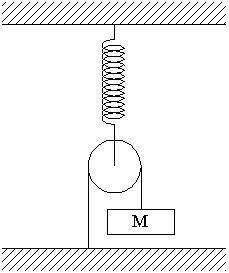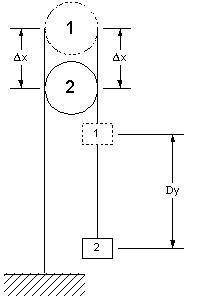# Pulley on a Spring

Jilvin

## Homework Statement

A pulley containing only a single mass, m, is hanging vertically from a mass with spring constant k. Find the period of vertical oscillation for the mass. Explanation in terms of energy and energy conservation is preferred.

## Homework Equations

Ay, there's the rub. You are supposed to find the spring's potential energy in terms of y (the height of the mass) and then use T=2*pi√(m/k) once you find the "true" k that corresponds with the mass (the second derivative of the potential energy U)

## The Attempt at a Solution

None worth noting. I know for sure that all of my attempts are terrible. If you need a closer look its from the lightandmatter.com textbook "Simple Nature", Chapter 2, question #35.

## Answers and Replies

You can find time period from purely energy method.
This is how:
Initial energy at mean position=potential energy stored at spring=0.5*k*d^2
Where d is extension of spring at mean position(mg/k).This term is constant.
Now write energy at any position=potential energy stored + kinetic energy of block=(0.5*k*x^2)+(0.5*m*v^2)
Final energy = Initial energy.
Differentiate both sides with respect to t
As Initial energy is constant so RHS becomes zero.
In LHS while differentiating we know that d/dt(x)=v and d/dt(v)=a(acceleration)
You get kx+ma=0
Thus a=-(k/m)*x
Thus proving it to be SHM.
Also a=-w^2*x
where w is omega(angular velocity) which is equal to (2*pi)/T.Where T is time period
Thus T=2*pi*(m/k)^0.5

Mentor

## Homework Statement

A pulley containing only a single mass, m, is hanging vertically from a mass with spring constant k. Find the period of vertical oscillation for the mass. Explanation in terms of energy and energy conservation is preferred.

## Homework Equations

Ay, there's the rub. You are supposed to find the spring's potential energy in terms of y (the height of the mass) and then use T=2*pi√(m/k) once you find the "true" k that corresponds with the mass (the second derivative of the potential energy U)

## The Attempt at a Solution

None worth noting. I know for sure that all of my attempts are terrible. If you need a closer look its from the lightandmatter.com textbook "Simple Nature", Chapter 2, question #35.

Your description doesn't quite catch the full flavor of the setup. Perhaps this diagram will help:You should post at least one of your attempts, or at least describe your assumptions and approach.

#### Attachments

Jilvin
Okay, here's my approach:

I have to find the potential energy of the spring *with respect* to the object that is oscillating, which is the mass on a pulley. I think the previous user may have solved for the simple case of a mass on a vertical spring. The mass is also on a *pulley*, and gneill has kindly provided a picture of the situation which is correct.

Roughly, what I've been trying to do is find the "x" term in (1/2)kx^2 in terms of the "y". I have tried (1/2)kx^2 + mgy = U, but I have no clue where to go from here. My goal is to obtain a reasonable second derivative of U to act as an effective "k" term to plug into T = 2$\pi$√(m/k) (the original k describing the spring's tension will not do unless the mass is tied directly to the end of the spring, which it isn't).

Im sorry. This is all I've got.

Mentor
It might be worthwhile analyzing the system for the sizes of motions that occur (if the mass moves by Δy, what is the corresponding displacement Δx of the spring?). And if the mass is creating a tension T in the rope, what is the tension transmitted to the spring? Can these characteristics be translated into an equivalent spring-mass system without a pulley by changing the spring constant?

Jilvin
Yeah, that's what I was thinking because one of the problems is in a similar flavor. Finding the increment in y compared to the increment in the spring displacement is exactly what I am looking for. I'm totally at a dead end with this.

Mentor
The total length of the rope is constant. Draw a picture with the pulley at two different heights. Determine how much rope has to pass over the pulley with that change in height.